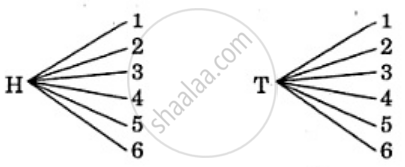Department of Pre-University Education, KarnatakaPUC Karnataka Science Class 11
Advertisement Remove all ads

# A Coin is Tossed and Then a Die is Thrown. Describe the Sample Space for this Experiment. - Mathematics

A coin is tossed and then a die is thrown. Describe the sample space for this experiment.

Advertisement Remove all ads

#### Solution

When a coin is tossed, the outcomes are H, T. When a dice is thrown, the outcomes can be 1, 2, 3, 4, 5, 6.
Thus, we can write the two parts of this experiment as follows:Hence, the sample space is given by
S = {(H, 1), (H, 2), (H, 3), (H, 4), (H, 5), (H, 6),
(T, 1), (T, 2), (T, 3), (T, 4), (T, 5), (T, 6)}

Is there an error in this question or solution?
Advertisement Remove all ads

#### APPEARS IN

RD Sharma Class 11 Mathematics Textbook
Chapter 33 Probability
Exercise 33.1 | Q 7 | Page 6
Advertisement Remove all ads

#### Video TutorialsVIEW ALL 

Advertisement Remove all ads
Share
Notifications

View all notifications

Forgot password?